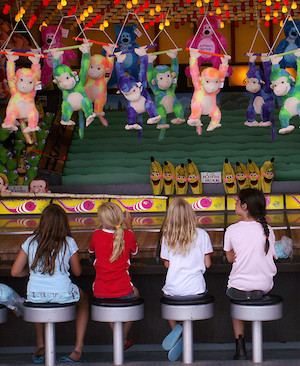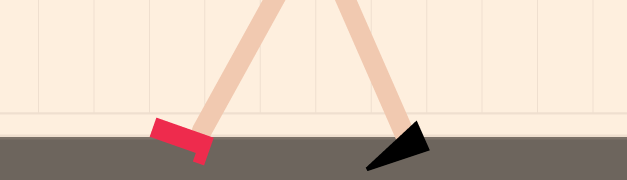Probability

# Permutations: Level 4 Challenges

How many 5 digit numbers can be formed if either
(a) every successive digit exceeds its predecessor, or
(b) every successive digit is smaller than its predecessor.

Detail: Leading 0's don't count, aka 04521 is not a 5-digit number.In how many ways can 4 boys (A, B, C, D) and 4 girls (W, X, Y, Z) be seated in a row, such that all the girls do not sit together?

Suppose $3$ married couples are randomly seated around a circular table. (It is not required that the members of any couple must be seated together.) The probability that no spouses are seated next to one another is $\dfrac{a}{b}$, where $a$ and $b$ are positive coprime integers. Find $a + b$.Alice, Benedict, Calvin, Dennis and Evelyn attended a party. At the end of the night, they each randomly grabbed a left shoe and a right shoe. What is the probability that each person left with exactly one of their own shoes?

Note: If the probability is 1%, enter it as 0.01.

$\huge INTERMEDIATE$ How many words can be made with the letters of the word $INTERMEDIATE$ if the order of vowels doesn't change ?

The "order of the vowels" refers to the sequence in which they occur. IE the sequence should be $I - E - E - I - A - E$.

×